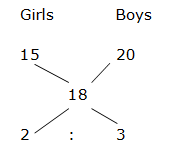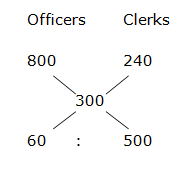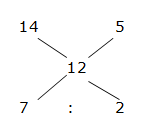# Quantitative Aptitude Questions (Averages) for SBI Clerk 2018 Day- 121

Dear Readers, SBI is conducting Online preliminary Examination for the recruitment of Clerical Cadre. preliminary Examination of SBI Clerk was scheduled from June 2018. To enrich your preparation here we have providing new series of Averages- Quantitative Aptitude Questions. Candidates those who are appearing in SBI Clerk Prelims Exam can practice these Quantitative Aptitude average questions daily and make your preparation effective.

[WpProQuiz 2587]

Click “Start Quiz” to attend these Questions and view Solutions

1. The average age of girls in the class is 15 years. The average age of boys in the class also added, then the average becomes 18. If there are 18 boys in the class and the average age of boys in the class is 20, then find how many girls in the class?
1. 18
2. 24
3. 12
4. 16
5. None of these
1. The average marks of Raji in a certain examination is 85. If she got 20 more marks in Maths and 12 more marks in English, then the average becomes 89. Find the total number of subjects she studied?
1. 8
2. 5
3. 7
4. 9
5. None of these
1. The average salary of the whole employees in a company is Rs. 300 per day. The average salary of officers is Rs. 800 per day and that of clerks is Rs. 240 per day. If the number of officers is 30, then find the number of clerks in the company?
1. 180
2. 160
3. 220
4. 250
5. None of these
1. The average age of 25 students in a class is 20. If the age of a teacher is included, then the average age increased by one year, find the age of the teacher?
1. 42 years
2. 46 years
3. 38 years
4. 34 years
5. None of these
1. The average height of 20 students is 105 cm. But later it was found that, the heights of 2 students were wrongly entered as 75 instead of 68 and 91 instead of 108. Then find the correct average?
1. 98 cm
2. 84 cm
3. 5 cm
4. 5 cm
5. None of these
1. The Average marks obtained by 45 students in an examination is 12. If the average marks of passed students are 14 and that of failed students are 5, then find the number of students who passed the examination?
1. 35
2. 42
3. 30
4. 26
5. None of these
1. The average weight of 20 people is increased by 2.2 kg when one man weight 53 kg is replaced by another man. Find the weight of new man?
1. 88 kg
2. 82 kg
3. 93 kg
4. 97 kg
5. None of these
1. Average number of sweets distributed in a class of 52 students is 5. If some number of students newly joined in the class and the average becomes 4, then find the number of newly joined students?
1. 18
2. 13
3. 22
4. 24
5. None of these
1. The average weight of 50 students in a class is 60 kg. The weights of two students were wrongly entered as 74 kg and 66 kg instead of 66 kg and 54 kg respectively. Find the corrected average weight of the class?
1. 58 kg
2. 50 kg
3. 60 kg
4. 80 kg
5. 20 kg
1. The average of 5 numbers is 120. The average of the first two numbers is 125 and the average of the last two numbers is 130. What is the third number?
1. 90
2. 110
3. 100
4. 125
5. None of these

Let the total number of girls in the class be x,

15x + (20*18) = (x+18)*18

15x+360 = 18x+324

36 = 3x

X =12

Total girls in the class is 12

Shortcuts:3’s = 18

1’s = 6

Total number of girls in the class = 2’s = 12

Let the total number of subjects be x,

Average = Sum/n

(85x + 20 + 12)/x = 89

85x + 32 = 89x

4x = 32

X = 8

Total number of subjects = 8

Let the number of clerks in the company be x,

800*30 + 240*x = 300(30 + x)

24000 + 240x = 9000 + 300x

15000 = 60x

X = 15000/60 = 250

Total number of clerks in the company = 250

Shortcuts:= > 3: 25

3’s = 30

1’s = 10

Total number of clerks in the company = 25’s = 250

Total age of 25 students = 25*20 = 500

Total age of 25 students and teacher = 26*21 = 546

Age of the teacher= 546 – 500 = 46 years

Shortcut:

N       Average

25      20

26      21

If both n and Average increased by 1, then the age of the teacher is,

= > (25 + 21) or (26 + 20) = 46 years

Correct Average = [(20*105) – (75 + 91) + (68 + 108)]/20

= > 105.5 cm

Let the number of passed students be x,

45*12 = 14x + (45 – x)*5

540 = 14x + 225 – 5x

540 – 225 = 9x

9x = 315

X = 35

Total number of passed students = 35

Shortcut:= > 7: 2

9’s = 45

1’s = 5

Total number of passed students = 7’s = 35

Average = 2.2, Number of people (n) = 20

Average = Total sum/n

Total weight increased = 2.2*20 = 44 kg

Weight of new man = 44 + 53 = 97 kg

Let the number of newly joined students be x,

According to the question,

52*5 = (52 + x)*4

260 = 208 + 4x

52 = 4x

X = (52/4) = 13

Total weight of 50 students = 50*60

New weight = (50*60) – 74 – 66 + 66 + 54 = (50*60) – 20

New average = (3000 – 20)/50 = 59.60 kg

Sum of 5 numbers = 120*5 = 600

Sum of first two numbers = 125*2 = 250

Sum of last two numbers = 130*2 = 260

Third number = 600 – (250 + 260)

= > 600 – 510 = 90

Daily Practice Test Schedule | Good Luck

 Topic Daily Publishing Time Daily News Papers & Editorials 8.00 AM Current Affairs Quiz 9.00 AM Quantitative Aptitude “20-20” 11.00 AM Vocabulary (Based on The Hindu) 12.00 PM General Awareness “20-20” 1.00 PM English Language “20-20” 2.00 PM Reasoning Puzzles & Seating 4.00 PM Daily Current Affairs Updates 5.00 PM Data Interpretation / Application Sums (Topic Wise) 6.00 PM Reasoning Ability “20-20” 7.00 PM English Language (New Pattern Questions) 8.00 PM